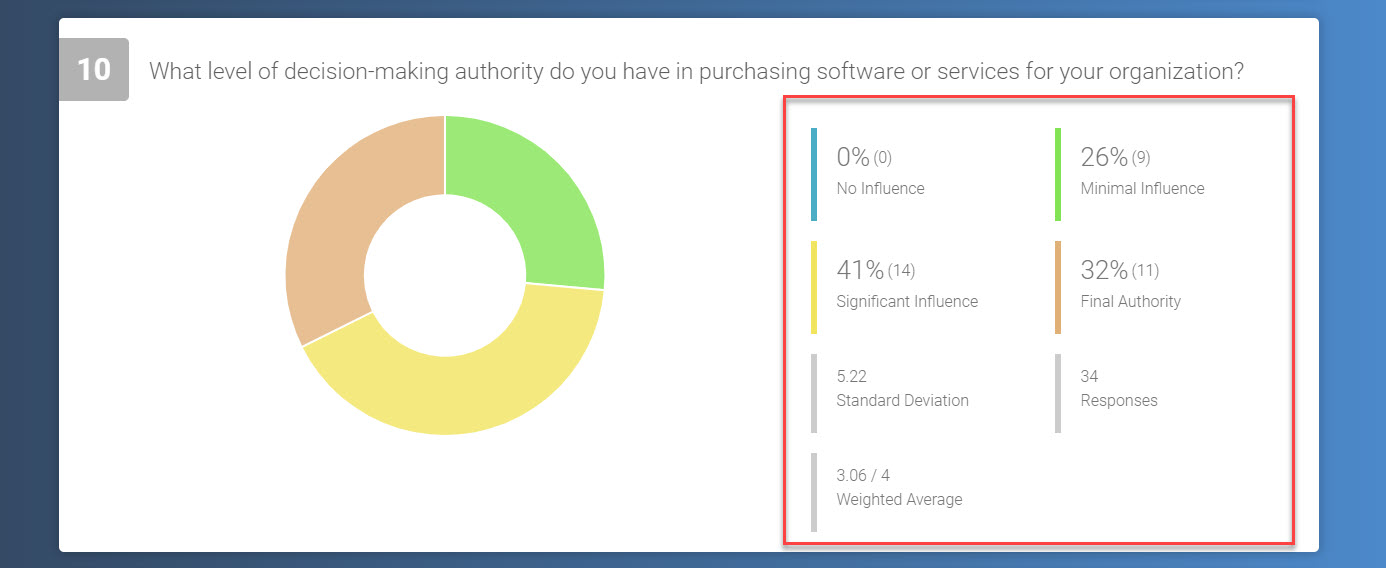Your project results will be collated into data tables for each question, which can be found under the Quick Report in the Results section.

This table will present you with averaged results from all participants who completed that question. You will also be provided with a possible three sets of statistics:• Standard Deviation
• Responses
• Weighted Average

Note: These statistics will not be provided for all question types, as some answers can’t be analyzed numerically.

### Standard Deviation

Standard deviation is calculated in contrast to the expected mean for a question. A lower standard deviation indicates that most numbers are close to the expected average, whilst a high standard deviation means the numbers chosen were more spread out.

### Responses

This number represents the total number of responses you received for a question.

### Weighted Average

This average is determined by the importance of individual figures within a data set. The higher percentage of times a number or answer is chosen by respondents, the more influential it becomes in calculating a weighted average.